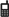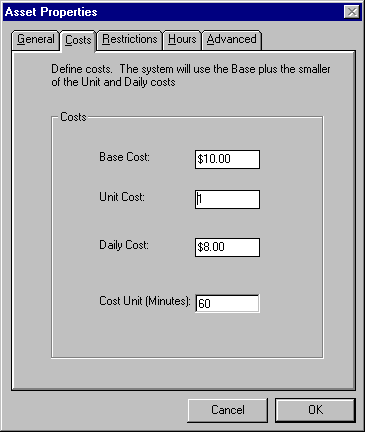(626)810-8031

19510 Buggywhip Circle, Walnut, CA 91789

# Asset CostEach asset can be given a base, unit, and daily cost. The net cost of a reservation is the base cost plus the unit times the number of units or the daily cost times the number of days. In the example here the location would cost \$10 plus \$1 per hour up to a maximum of 8 hours. Therefore: A 4 hour reservation would be \$14 A 4 hour, 30 minute reservation would be \$15 A 10 hour reservation would be \$18 In addition each organization and event type can be given a billing factor that represents a discount or surcharge on these rates.